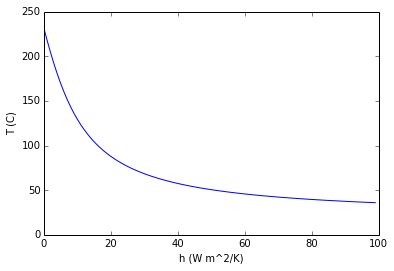Introduction¶

Example 1.1 Page 5¶

In :
L=.15;   		 			#[m] - Thickness of conducting wall
delT = 1400. - 1150.;  		#[K] - Temperature Difference across the Wall
A=.5*1.2; 					#[m^2] - Cross sectional Area of wall = H*W
k=1.7;    					#[W/m.k] - Thermal Conductivity of Wall Material
#calculations
#Using Fourier's Law eq 1.2
Q = k*delT/L;    			#[W/m^2] - Heat Flux

q = A*Q;          			#[W] - Rate of Heat Transfer
#results
print '%s %.2f %s' %("\n \n Heat Loss through the Wall =",q," W");
#END

Heat Loss through the Wall = 1700.00  W

Example 1.2 Page 11¶

In :

import math
d=.07;    									#[m] - Outside Diameter of Pipe
Ts = 200+273.15;  							#[K] - Surface Temperature of Steam
Tsurr = 25+273.15; 							#[K] - Temperature outside the pipe
e=.8; 										#  Emissivity of Surface
h=15;    									#[W/m^2.k] - Thermal Convectivity from surface to air
stfncnstt=5.67*math.pow(10,(-8));   	 	# [W/m^2.K^4] - Stefan Boltzmann Constant
#calculations
#Using Eq 1.5
E = e*stfncnstt*Ts*Ts*Ts*Ts;    			#[W/m^2] - Emissive Power
G = stfncnstt*Tsurr*Tsurr*Tsurr*Tsurr;    	#[W/m^2] - Irradiation falling on surface
#results
print '%s %.2f %s' %("\n (a) Surface Emissive Power = ",E," W/m^2");
print '%s %.2f %s' %("\n     Irradiation Falling on Surface =",G," W/m^2");

#Using Eq 1.10 Total Rate of Heat Transfer Q  = Q by convection + Q by radiation
q = h*(math.pi*d)*(Ts-Tsurr)+e*(math.pi*d)*stfncnstt*(Ts*Ts*Ts*Ts-Tsurr*Tsurr*Tsurr*Tsurr);          #[W]

print '%s %.2f %s' %("\n\n (b) Total Heat Loss per unit Length of Pipe=",q," W");
#END
(a) Surface Emissive Power =  2273.36  W/m^2

Irradiation Falling on Surface = 448.05  W/m^2

(b) Total Heat Loss per unit Length of Pipe= 998.38  W

Example 1.4 Page 20¶

In :
import math
Ts = 56.4+273.15;  					#[K] - Surface Temperature of Steam
Tsurr = 25+273.15; 					#[K] - Temperature of Surroundings
e=.88; 								#  Emissivity of Surface

#As h=(10.9*math.pow(V,.8)[W/m^2.k] - Thermal Convectivity from surface to air
stfncnstt=5.67*math.pow(10,(-8)); 	# [W/m^2.K^4] - Stefan Boltzmann Constant

A=2*.05*.05;    					# [m^2] Area for Heat transfer i.e. both surfaces

E = 11.25;  			 	 		#[W] Net heat to be removed by cooling air
#calculations

#Using Eq 1.10 Total Rate of Heat Transfer Q  = Q by convection + Q by radiation
Qconv = E - Qrad;					#[W]

#As Qconv = h*A*(Ts-Tsurr) & h=10.9 Ws^(.8)/m^(-.8)K.V^(.8)

V = math.pow(Qconv/(10.9*A*(Ts-Tsurr)),(1/0.8));
#results

print '%s %.2f %s' %("\n\n Velocity of Cooling Air flowing= ", V,"m/s");
#END
EXAMPLE 1.4   Page 20

Velocity of Cooling Air flowing=  9.40 m/s

Example 1.6 Page 26¶

In :
import math
A=1.8;	    								# [m^2] Area for Heat transfer i.e. both surfaces
Ti = 35+273.; 	 							#[K] - Inside Surface Temperature of Body
Tsurr = 297.; 								#[K] - Temperature of surrounding
Tf = 297.; 									#[K] - Temperature of Fluid Flow
e=.95; 										#  Emissivity of Surface
L=.003;    									#[m] - Thickness of Skin
k=.3;  										#  Effective Thermal Conductivity
h=2;    									#[W/m^2.k] - Natural Thermal Convectivity from body to air
stfncnstt=5.67*math.pow(10,(-8)); 			# [W/m^2.K^4] - Stefan Boltzmann Constant
#Using Eq 1.5

Tsa=305.;  			    				    #[K]  Body Temperature Assumed
#calculations

Ts=307.19
q = k*A*(Ti-Ts)/L;          #[W]

print '%s' %("\n\n (I) In presence of Air")
print '%s %.2f %s' %("\n (a) Temperature of Skin = ",Ts,"K");
print '%s %.2f %s' %("\n (b) Total Heat Loss = ",q," W");

#When person is in Water
h = 200;    								#[W/m^2.k] - Thermal Convectivity from body to water
hr = 0;    									# As Water is Opaque for Thermal Radiation
Ts = (k*Ti/L + (h+hr)*Tf)/(k/L +(h+hr));   	#[K]  Body Temperature
q = k*A*(Ti-Ts)/L;          				#[W]
#results

print '%s' %("\n\n (II) In presence of Water")
print  '%s %.2f %s' %("\n (a) Temperature of Skin =",Ts," K");
print  '%s %.2f %s' %("\n (b) Total Heat Loss =",q," W");

#END

(I) In presence of Air

(a) Temperature of Skin =  307.19 K

(b) Total Heat Loss =  145.80  W

(II) In presence of Water

(a) Temperature of Skin = 300.67  K

(b) Total Heat Loss = 1320.00  W

Example 1.7 Page 30¶

In :
%matplotlib inline

import math
import numpy
from numpy import roots
import matplotlib
from matplotlib import pyplot
Tsurr = 30+273;                                     #[K] - Temperature of surrounding
Tf = 20+273;                                        #[K] - Temperature of Fluid Flow
e=.5;                                               #  Emissivity of Surface
a = .8;                                             # Absorptivity of Surface
G = 2000;                                           #[W/m^2] - Irradiation falling on surface
h=15;                                               #[W/m^2.k] - Thermal Convectivity from plate to air
stfncnstt=5.67*math.pow(10,(-8));                   # [W/m^2.K^4] - Stefan Boltzmann Constant
T=375;                                              #[K] Value initially assumed for trial-error approach
#Using Eq 1.3a & 1.7 and trial-and error approach of Newton Raphson
#calculations and results
while(1>0):
f=((a*G)-(h*(T-Tf)+e*stfncnstt*(T*T*T*T - Tsurr*Tsurr*Tsurr*Tsurr)));
fd=(-h*T-4*e*stfncnstt*T*T*T);
Tn=T-f/fd;
if(((a*G)-(h*(Tn-Tf)+e*stfncnstt*(Tn*Tn*Tn*Tn - Tsurr*Tsurr*Tsurr*Tsurr)))<.01):
break;
T=Tn;

print '%s %.2f %s' %("\n (a) Cure Temperature of Plate =",T-273.,"degC\n");
#solution (b)
Treq=50+273;
#def T(h):
#    t=375;
#    while(1>0):
#        f=((a*G)-(h*(t-Tf)+e*stfncnstt*(t*t*t*t - Tsurr*Tsurr*Tsurr*Tsurr)));
#        fd=(-h*t-4*e*stfncnstt*t*t*t);
#        Tn=t-f/fd;
#        if((a*G)-(h*(Tn-Tf)+e*stfncnstt*(Tn*Tn*Tn*Tn - Tsurr*Tsurr*Tsurr*Tsurr))<.01):
#           break;
#    tnew=Tn;
#    return tnew;

def T(h):
global rt
coeff = ([-e*stfncnstt, 0,0, -h, a*G+h*Tf+e*stfncnstt*Tsurr*Tsurr*Tsurr*Tsurr]);
rot=numpy.roots(coeff);
rt=rot;
#for i in range (0,3):
#    if 273<rot[i]<523:
#        rt=rot[i];
return rt

h = range(0,100)
tn=range(0,100)
for i in range (0,100):
tn[i] = T(i) -273;

Ti=50+273;
hnew=((a*G)-(e*stfncnstt*(Ti**4 - Tsurr**4)))/(Ti-Tf);

pyplot.plot(h,tn);
pyplot.xlabel("h (W m^2/K)");
pyplot.ylabel("T (C)");
pyplot.show();
print '%s %.2f %s' %("\n (b) Air flow must provide a convection of =",hnew," W/m^2.K");
print '%s' %("\n The code for the graph requires more than 10 min to run. ")
print '%s' %("\n To run it, please remove comments. It is perfectly correct. The reason it takes such a long time")
print '%s' %("\n  is that it needs to calculate using Newton raphson method at 100 points. Each point itself takes a minute.")
#END
Populating the interactive namespace from numpy and matplotlib

(a) Cure Temperature of Plate = 104.30 degC

WARNING: pylab import has clobbered these variables: ['f', 'e']
%pylab --no-import-all prevents importing * from pylab and numpy(b) Air flow must provide a convection of = 51.01  W/m^2.K

The code for the graph requires more than 10 min to run.

To run it, please remove comments. It is perfectly correct. The reason it takes such a long time

is that it needs to calculate using Newton raphson method at 100 points. Each point itself takes a minute.
In [ ]: